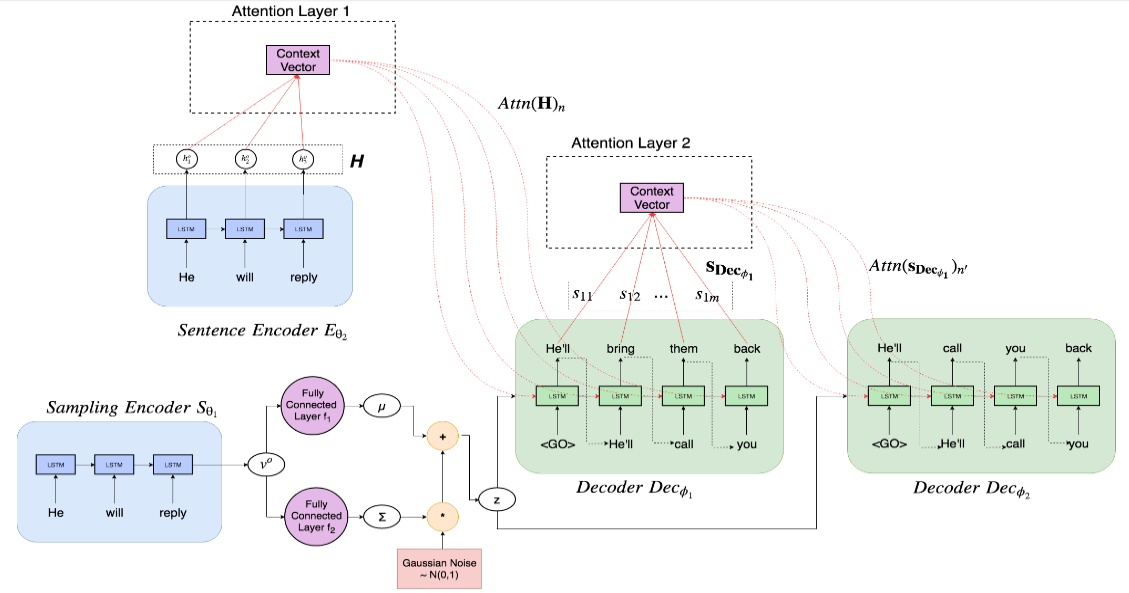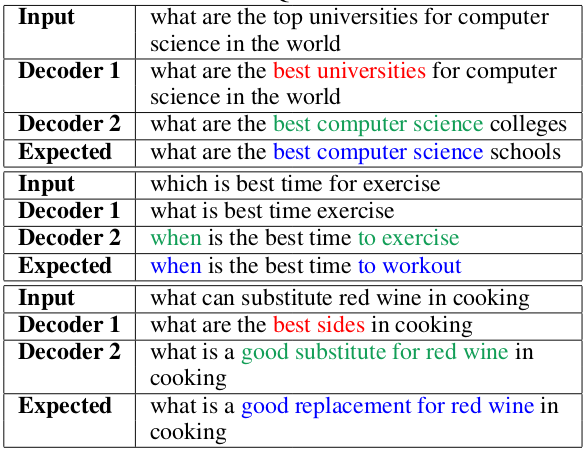# 论文笔记： ReDecode Framework for Iterative Improvement in Paraphrase Generation

## 作者

• Milan Aggarwal
• Nupur Kumari
• Ayush Bansal
• Balaji Krishnamurthyk

## 观点与事实

• 当前的一些序列生成方法都是一锤子买卖，在生成的时候改正错误的能力太差，生成之后也不能去改进生成质量
• 人类对话中用复杂的复述来表达相同意图的行为很普遍，但对机器来说要辨别或生成复述句是很困难的
• VAE 学习的是一个概率分布，所以用于生成式的任务很合适

## 相关工作

• 1983. Paraphrasing questions using givenand new information
• 2003. Learning to paraphrase: anunsupervised approach using multiple-sequence alignment
• 2004. Monolingualmachine translation for paraphrase generation. Associationfor Computational Linguistics.
• 2004. Synonymous para-phrasing using wordnet and internet
• 2016. Neural paraphrase genera-tion with stacked residual lstm networks
• 2017. Learn-ing paraphrastic sentence embeddings from back-translatedbitext
• 2017. Joint copying andrestricted generation for paraphrase
• 2017. Learning to paraphrase for question answering
• 2017. Adeep generative framework for paraphrase generation
• 2018. Adversarial example generation with syn-tactically controlled paraphrase networks

## 模型/方法/结论• Sampling Encoder

这个 Encoder 用来根据输入句子 $$x_{o}$$ 产生一个概率分布，然后从 x 中进行采样得到一个 latent vector $$z$$

在这篇论文里，Sampling Encoder 由一个单层的 LSTM 和两个全连接层构成。

首先输入句子 $$x_{o}$$ 经过 LSTM 后得到整个句子的向量表示 $$v_{o}$$，然后分别输入一个全连接层，得到期望概率分布的均值 $$\mu$$ 和方差 $$\sum$$，用这个均值和方差就得到了一个正态分布 $$N(\mu, \sum)$$，从这个概率分布中采样得到最终需要的 $$z$$。

为了让最终模型的输出能更有多样性，在训练的时候，作者对 $$\mu$$ 和 $$\sum$$ 施加高斯噪声扰动来得到不同的 $$z$$。

• Sentence Encoder

这个 Encoder 用来对得到输入句子 x 的语义表示，用来作为 decoder 的语义输入。

这个 Encoder 就比较简单了，一个两层的 LSTM，输出 hidden state 序列 $$H={h_{1}^{o}, h_{2}^{o}...h_{n}^{o}}$$，并在 Decoder 中对其进行 attention 计算。

• Sequencd Decoders

这个是最终用来生成的复述句的，也是整篇论文中的要点。

和常规的一个 decoder 做法不一样的是，作者认为生成的部分，应该用多个 decoder 来迭代地生成结果。具体来说，这篇论文的做法是这样的

• 第一个 decoder 以 Sentence Encoder 输出的 hidden state 向量 $$H$$ 和 Sampling Encoder 输出的 latent vector $$z$$ 作为输入

$$p_{1}=Dec_{\phi_{1}}(z, Attn(H))$$

• 随后的 decoder 则以前一个 decoder 的输出和 Sampling Encoder 输出的 $$z$$ 作为输入

$$p_{i}=Dec_{\phi_{i}}(z, Attn({s_{Dec_{\phi_{i-1}}}}))$$## 总结

VAE 的部分我其实并不太熟悉，需要去补课。至于 Decoder 的部分，这种迭代生成的路子，其实早就见过了，比如说 HMN 模型，以及一些在 attention 机制上的改进工作之类的。